# ID3 Algorithm Decision Trees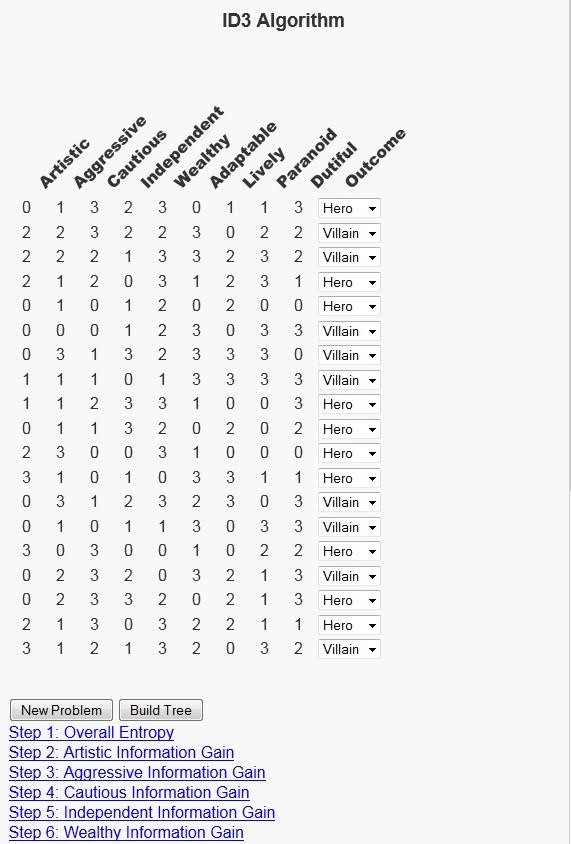As I grow LEARNINGlover.com, I’m always thinking of different ways to expose my own personality through the site. This is partially because it is easier for me to talk about subjects where I am already knowledgeable, but it is more-so being done to help make some of these algorithms and concepts I encode more understandable, and sometimes relating foreign concepts to everyday life makes them easier to understand.

Today, I’d like to write about decision trees, and the ID3 algorithm for generating decision trees in particular. This is a machine learning algorithm that builds a model from a training data set consisting of a feature vector and an outcome. Because our data set consists of an outcome element, this falls into the category of supervised machine learning.

The model that the ID3 algorithm builds is called a decision tree. It builds a tree based on the features, or columns of the data set with a possible decision corresponding to each value that the feature can have. The algorithm selects the next feature by asking “which feature tells me the most about our data set?” This question can be answered first by asking how much “information” is in the data set, and then comparing that result with the amount of information in each individual feature.

In order to execute this algorithm we need a way to measure both the amount the information in outcomes of the overall data set as well as how much each feature tells us about the data set. For the first, we will use entropy, which comes from the field of information theory and encoding. Entropy is based on the question of how many bits are necessary to encode the information in a set. The more information, the higher the entropy, and the more bits required to encode that information. Although we are not encoding, the correlation between high information and high entropy suits our purposes.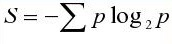To understand how much each feature tells us about the outcomes of the data set we will build on the concept of entropy to define the information gain of a feature. Each feature has multiple options, so the dataset can be partitioned based on each possible value of this feature. Once we have this partition, we can calculate the entropy of each subset of the rows of data. We define the information gain of a feature as the sum over all possible outcomes of that feature can have of the entropy of that outcome multiplied by the probability of that outcome.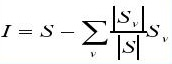To illustrate this algorithm, I decided to relate it to the question of whether we think of a character in a novel as a hero or villain. This is interesting because I try to read at least one book a month and as I’m reading, I often find myself asking this question about characters based on the traits of the characters as well as characters I’ve read about. In order to build an interactive script for this problem, I considered 25 possible character traits that could be present. A subset of these 25 character traits will be selected and a row will be generated grading a fictional character on a scale of 0 to 3 (0 meaning that they do not possess the trait at all, 3 meaning that the trait is very strong in their personality), and users will be asked whether they think a character with the given character traits should be listed as a hero or a villain. Then there is a button at the bottom of the script with the text “Build Tree” that executes the ID3 Algorithm and shows a decision tree that could be used to reach the set of decisions given by the user.

The possible features are:
Abstract, Adaptable, Aggressive, Ambition, Anxiety, Artistic, Cautious, Decisive, Honesty, Dutiful, Fitness, Intellect, Independent, Introverted, Lively, Open-minded, Orderly, Paranoid, Perfectionist, Romantic, Sensitive, Stable, Tension, Warmth and Wealthy

Once users select the option to build the tree, there will be several links outlining each step in the process to build this tree. These links will allow for users to expand the information relating to that step and minimize that information when done. Hopefully this will help users to understand each step more. I must say that as much fun as it has been writing this program, there were several questions when trying to explain it to others. Hopefully users get as much fun from using this tool as I had in creating it. As always feel free to contact me with any comments and or questions.

Ok, so here’s a link to the ID3 Algorithm Page. Please check it out and let me know what you think.

# A JavaScript Implentation of MapReduce’s WordCountYou can view a javascript implementation of the WordCound Program in Mapreduce at Javascript Implementation of Mapreduce WordCount

One of the big things in the world of Data Science and Cloud Computing is the map-reduce implementation of various algorithms. This is not always a straightforward procedure and so learning to think in terms of map-reduce implementations can be a challenging conversion from thinking in a functional programming frame of mind. In light of this I thought it would be convenient to try to help users “visualize” this concept. This is a challenging task because there are many concepts of cloud computing that I am unable to provide in this environment. However, just as many of the books on MapReduce provide pseudo-code on various implementations of algorithms in a Map-Reduce environment, I will attempt to show how data flows from the input to the mappers to the shuffle and sort phase to the reducers and finally to generate the output. I leave the users the task of actually putting these into the context of a Java MapReduce environment.

I want to first speak about the concept of (key, value) pairs which is a very important in MapReduce programming. I will speak about this in the context of a WordCount program. The purpose of a WordCount program is to count the number of occurrences of each word in a given file. First data is input to the mapper in (key, value) pairs. For our example, the key will be the line number of input (so each line of input will go to a different mapper) and the value will be the text present on that line. Once the mapper has the input, it will perform some operation on it and output data again in (key, value) pairs. In the WordCount example, the mappers will simply output each word that occurs as a new key on that line and the integer “1” as the associated value (note that a single mapper can output multiple (key, value) pairs).

One of the main things to understand in a MapReduce is that there are a number of Mappers running on a given input file and these Mappers cannot interact with one another. Suppose we have two different mappers, lets call them Mapper1 and Mapper2 that are each working on two different lines of input from a file. If both lines of input have the word “apple”, there is no way for Mapper2 to know that Mapper1‘s line of input also has this word. In this setting that’s perfectly fine because the Shuffle and Sort phase is where all the (key, value) pairs that were output by the mappers, compares the keys to one another and if they are equal to one another combines their respective values into a list of values. Unequal keys are sorted.

So if both Mapper1 and Mapper2 contained the word “apple” in their line of text, then the (key, value) pair (apple, 1) will occur twice. So the Shuffle and Sort phase will notice this and output the (key, value) pair (apple, {1, 1}).

Each reducer is then given a key and a list of values that were output by the mappers. The goal will be to perform some operation and again output data in (key, value) pairs. In the WordCount example, we will use what is known as the sumReducer. It gets this name because its job is simply to sum the values in the list of values and output the (key, value) pair that is the original key and this sum of values.

You can view a javascript implementation of this at Javascript Implementation of Mapreduce WordCount

# Hidden Markov Models: The Baum-Welch Algorithm

Suppose you are at a table at a casino and notice that things don’t look quite right. Either the casino is extremely lucky, or things should have averaged out more than they have. You view this as a pattern recognition problem and would like to understand the number of ‘loaded’ dice that the casino is using and how these dice are loaded. To accomplish this you set up a number of Hidden Markov Models, where the loaded die are the latent (hidden) variables, and would like to determine which of these, if any is more likely to be using.

First lets go over a few things.

We will call each roll of the dice an observation. The observations will be stored in variables o1, o2, …, oT, where T is the number of total observations.

To generate a hidden Markov Model (HMM)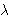we need to determine 5 parameters:

• The N states of the model, defined by S = {S1, …, SN}
• The M possible output symbols, defined by= {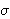1,2, …,M}
• The State transition probability distribution A = {aij}, where aij is the probability that the state at time t+1 is Sj, given that the state at time t is Si.
• The Observation symbol probability distribution B = {bj(k)} where bj(k) is the probability that the symbolk is emitted in state Sj.
• The initial state distribution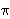= {i}, wherei is the probability that the model is in state Si at time t = 0.

The HMMs we’ve generated are based on two questions. For each question, you have provided 3 different answers which leads to 9 possible HMMs. Each of these models has its corresponding state transition and emission distributions.

• How often does the casino change dice?
• 0) Dealer Repeatedly Uses Same Dice
• 1) Dealer Uniformly Changes Die
• 2) Dealer Rarely Uses Same Dice
• Which sides on the loaded dice are more likely?
• 0) Larger Numbers Are More Likely
• 1) Numbers Are Randomly Likely
• 2) Smaller Numbers Are More Likely
How often does the casino change dice?
Which sides on
are more likely?
 (0, 0) (0, 1) (0, 2) (1, 0) (1, 1) (1, 2) (2, 0) (2, 1) (2, 2)

One of the interesting problems associated with Hidden Markov Models is called the Learning Problem, which asks the question “How can I improve a HMMso that it would be more likely to have generated the sequence O = o1, o2, …, oT?

The Baum-Welch algorithm answers this question using an Expectation-Maximization approach. It creates two auxiliary variables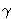t(i) and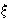t(i, j). The variablet(i) represents the probability of being in state i at time t, given the entire observation sequence. Likewiset(i, j) represents the joint probability of being in state i at time t and of being in state j at time t+1, given the entire observation sequence. They can be calculated byt(i) = (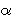t(i) *t(i) )j = 1 to N(t(j) *t(j))

andt(i, j) = (t(i) * ai, j *t+1(j) * bj(ot+1) )i’ = 1 to Nj’ = 1 to N(t(i’) * ai’, j’ *t+1(j’) * bj’(ot+1) )

As you can see, these are a direct result of calculations offrom the Forward algorithm andfrom the Backwards algorithm. Once we have calculated these variables, we can update the parameters of the model as follows: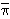i =1(i)i,j =t = 1 to T-1(t(i))t = t to T-1 (t(i, j))

// [b bar]_{j, k} = Sigma_{t = 1 to T, o_t = o_k} gamma_{t, j} / Sigma_{t = 1 to T} gamma_{t, j}, 1 <= j <= N, 1 <= k <= Mj(ok) =t = 1 to T-1, ot = okt(j)t = 1 to T-1t(j)

We can iterate this procedure a finite number of times or until it converges. This will generate a new model,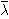= {N,,,,}.

There is more on this example at LEARNINGlover.com: Hidden Marokv Models: The Baum-Welch Algorithm.

Some further reading on Hidden Markov Models:

# Hidden Markov Models: The Viterbi Algorithm

I just finished working on LEARNINGlover.com: Hidden Marokv Models: The Viterbi Algorithm. Here is an introduction to the script.

Suppose you are at a table at a casino and notice that things don’t look quite right. Either the casino is extremely lucky, or things should have averaged out more than they have. You view this as a pattern recognition problem and would like to understand the number of ‘loaded’ dice that the casino is using and how these dice are loaded. To accomplish this you set up a number of Hidden Markov Models, where the loaded die are the latent variables, and would like to determine which of these, if any is more likely to be using.

First lets go over a few things.

We will call each roll of the dice an observation. The observations will be stored in variables o1, o2, …, oT, where T is the number of total observations.

To generate a hidden Markov Model (HMM)we need to determine 5 parameters:

• The N states of the model, defined by S = {S1, …, SN}
• The M possible output symbols, defined by= {1,2, …,M}
• The State transition probability distribution A = {aij}, where aij is the probability that the state at time t+1 is Sj, given that the state at time t is Si.
• The Observation symbol probability distribution B = {bj(k)} where bj(k) is the probability that the symbolk is emitted in state Sj.
• The initial state distribution= {i}, wherei is the probability that the model is in state Si at time t = 0.

The HMMs we’ve generated are based on two questions. For each question, you have provided 3 different answers which leads to 9 possible HMMs. Each of these models has its corresponding state transition and emission distributions.

• How often does the casino change dice?
• 0) Dealer Repeatedly Uses Same Dice
• 1) Dealer Uniformly Changes Die
• 2) Dealer Rarely Uses Same Dice
• Which sides on the loaded dice are more likely?
• 0) Larger Numbers Are More Likely
• 1) All Numbers Are Equally Likely
• 2) Smaller Numbers Are More Likely
How often does the casino change dice?
Which sides on
are more likely?
 (0, 0) (0, 1) (0, 2) (1, 0) (1, 1) (1, 2) (2, 0) (2, 1) (2, 2)

One of the interesting problems associated with Hidden Markov Models is called the Decoding Problem, which asks the question “What is the most likely sequence of states that the HMMwould go through to generate the sequence O = o1, o2, …, oT?

The Viterbi algorithm finds answers this question using Dynamic Programming. It creates an auxiliary variable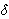t(i) which has the highest probability that the partial observation sequence o1, …, ot can have, given that the current state is i. This variable can be calculated by the following formula:t(i) = maxq1, …, qt-1 p{q1, …, qt-1, qt = i, o1, …, ot |}.1(j) =jbj(o1), for 1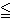jN.

Once we have calculatedt(j) we also keep a pointer to the max state. We can then find the optimal path by looking at arg max 1jNT(j) and then backtrack the sequence of states using the pointer.

There is more on this example at LEARNINGlover.com: Hidden Marokv Models: The Viterbi Algorithm.

Some further reading on Hidden Markov Models:

# Learning the Apriori AlgorithmI have finished a script that runs the Apriori algorithm.

When we are given a large set of transactions, we are often interested in discovering patterns inside these transactions. The Apriori algorithm provides a means for formulating what are known as “association rules” for the set of transactions. An association rule is an observation from the database between different items inside a transaction. For example, the statement “If a customer buys chips they are 60% more likely to also purchase dip” could be an association rule based on data from supermarket purchases. The number 60% is called the confidence we have in the rule and we are generally interested in rules with higher confidence.

The Apriori algorithm takes as input a transaction database and a “threshold”. The initial pass through the database performs a count on each single item in the database and checks how many transactions contain each item. The algorithm proceeds by the Apriori property which states that “any subset of a frequent itemset must also be frequent”. What this means is that when checking the subsets of length 2 (and greater), we can ignore those subsets that contain an element that does not meet the minimum support, as it cannot be a part of a frequent itemset.

So lets look at an example. Suppose our list of transactions for the items Chips, Dip, Soda, Napkins and Paper Plates are as follows:

(Chips, Dip, Soda, Napkins, Paper Plates)
1 1 0 1 0
0 0 0 1 0
1 1 1 0 1
0 1 1 0 1
1 0 0 1 0
1 0 0 0 1
1 1 1 0 1
0 0 1 0 1
1 0 0 0 0
1 0 1 1 0
0 1 1 1 0
1 1 0 0 0
1 0 1 1 0
0 0 0 1 1
1 1 1 1 0
0 0 0 0 0
0 0 0 0 0
1 1 1 0 0
0 0 0 1 1
1 1 1 0 1

And lets suppose that we’re interested in finding the collections that occur more than a quarter (25%) of the time.

What we see from simply summing the columns and dividing by the number of columns is that 60% of the people purchased chips, 45% purchased dip, 50% purchased soda, 45% purchased napkins, and 40% purchased paper plates. Since these are all above our minimum threshold, they are all possible as elements of future collections.

When looking at larger collections, we see that:
– 35% of the people purchased both chips and dip
– 35% of the people purchased both chips and soda
– 25% of the people purchased both chips and napkins
– 35% of the people purchased both dip and soda
– 25% of the people purchased both soda and paper plates
– no other size two collections are above the minimum threshold.

You’ll note that since only 20% of the people purchased chips and paper plates, it is not above the minimum threshold and so we can ignore it in future collections. From the set of size 2 itemsets, we can derive our list of size three itemsets.

In particular, we see that:
– 25% of the people purchased all three of chips, dip, and soda.
– no other size three collections are above the minimum threshold.

The hierarchy of the itemsets is displayed in the image above.

To play around with this algorithm and understand more of its properties, visit Apriori algorithm.

Other Blogs that have covered this topic:
Analytics and Visualization of Big Data
Statistical Research